### Home > MC2 > Chapter 5 > Lesson 5.1.2 > Problem5-22

5-22.
1. Translate each of these situations into a variable expression such as those found in a magic number chart. Homework Help ✎

1. Think of a number and multiply it by seven.

2. Think of a number and divide it by eight.

3. Think of a number and reduce it by ten.

4. Think of a number, add two, then multiply by five.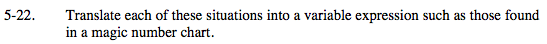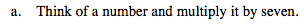Use x to represent any number you think of.

What would the expression look like if you multiplied your number by 7?

7x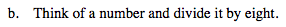See (a).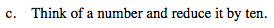What happens to a number when you reduce it by 10?

See (a).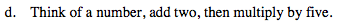See (a).

5 ( x + 2 )